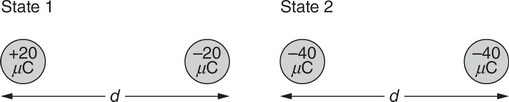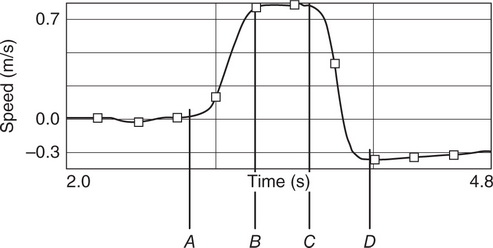# AP Physics 1 Practice Test 17

### Test Information10 questions18 minutes

1.Question below refers to the following information: A bicycle wheel of known rotational inertia is mounted so that it rotates clockwise around a vertical axis, as shown in the first figure. Attached to the wheel’s edge is a rocket engine, which applies a clockwise torque τ on the wheel for a duration of 0.10 s as it burns. A plot of the angular position θ of the wheel as a function of time t is shown in the graph.

In addition to the wheel’s rotational inertia and the duration of time the engine burns, which of the following information from the graph would allow determination of the net torque the rocket exerts on the wheel?

2.Question below refers to the following information: A bicycle wheel of known rotational inertia is mounted so that it rotates clockwise around a vertical axis, as shown in the first figure. Attached to the wheel’s edge is a rocket engine, which applies a clockwise torque τ on the wheel for a duration of 0.10 s as it burns. A plot of the angular position θ of the wheel as a function of time t is shown in the graph.

Which of the following graphs sketches the angular acceleration α of the wheel as a function of time?

3. A rock drops onto a pond with a smooth surface. A few moments later, the wave produced by the rock’s impact arrives at the shore, touching the ankles of a wading child. Which of the following observations provides evidence that the portion of the wave hitting the child’s ankles carries less energy than the wave did when it was first created by the rock’s impact?

4.Question below refer to the following information:

Two metal balls of equal mass (100 g) are separated by a distance (d). In state 1, shown in the figure, the left ball has a charge of +20 µC, while the right ball has a charge of –20 µC. In state 2, both balls have an identical charge of –40 µC.

Which of the following statements about the magnitudes of the electrostatic and gravitational forces between the two balls is correct?

5.Question below refer to the following information:

Two metal balls of equal mass (100 g) are separated by a distance (d). In state 1, shown in the figure, the left ball has a charge of +20 µC, while the right ball has a charge of –20 µC. In state 2, both balls have an identical charge of –40 µC.

An experimenter claims that he took the balls from state 1 to state 2 without causing them to contact anything other than each other. Which of the following statements provides correct evidence for the reasonability of this claim?

6. A guitar string creates a sound wave of known frequency. Which of the following describes a correct and practical method of measuring the wavelength of the sound wave with a meterstick?

7.When a 0.20 kg block hangs at rest vertically from a spring of force constant 4 N/m, the spring stretches 0.50 m from its unstretched position, as shown in the figure. Subsequently, the block is stretched an additional 0.10 m and released such that it undergoes simple harmonic motion. What is the maximum kinetic energy of the block in its harmonic motion?

8.Question below refers to the following information:

A student pushes cart A toward a stationary cart B, causing a collision. The velocity of cart A as a function of time is measured by a sonic motion detector, with the resulting graph shown in the figure.

At which labeled time did the collision begin to occur?

9.Question below refers to the following information:

A student pushes cart A toward a stationary cart B, causing a collision. The velocity of cart A as a function of time is measured by a sonic motion detector, with the resulting graph shown in the figure.

What additional measurements, in combination with the information provided in the graph, could be used to verify that momentum was conserved in this collision?

10. A car of mass m initially travels at speed v. The car brakes to a stop on a road that slants downhill, such that the car’s center of mass ends up a vertical height h below its position at the start of braking. Which of the following is a correct expression for the increase in the internal energy of the road-car system during the braking process?